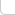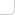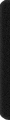# 某岛

… : "…アッカリ～ン . .. . " .. .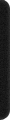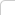June 27, 2022

## Problem D. Permutation Graph

const int N = int(5e5) + 9;
int a[N], n; PII l[N], r[N];

int f(PII s[][N], bool b, int x) {
int p = s[b][x].se; if (x == p) return 0;
return 1 + f(s, b^1, p);
}

int gao() {
RD(n); REP(i, n) RD(a[i]);
l = l = {a, 0};
FOR(i, 1, n) {
l[i] = min(l[i-1], {a[i], i});
l[i] = max(l[i-1], {a[i], i});
}
r[n-1] = r[n-1] = {a[n-1], n-1};
DWN(i, n-1, 0) {
r[i] = min(r[i+1], {a[i], i});
r[i] = max(r[i+1], {a[i], i});
}

int p = l[n-1].se;
return f(l, 1, p) + f(r, 0, p);
}

int main() {

#ifndef ONLINE_JUDGE
freopen("in.txt", "r", stdin);
//freopen("/Users/minakokojima/Documents/GitHub/ACM-Training/Workspace/out.txt", "w", stdout);
#endif

Rush OT(gao());
}


（这个结论实际上比做法 1 的结论弱。。。而且不用做法 1 的方式证。。也其实更麻烦。。。证明可以考察某个点 x，如果它向某个方向，例如右边，连接了 r0, r1, r2… 一组点，那么这些点一定构成一个递增或者递减序列。。因此自然 r 序列之间也互相连通，因此直接连到最远的点更优。）

2.1 栈版本

const int N = int(5e5) + 9;
int a[N], n; int r[N];

int gao() {
RD(n); REP(i, n) RD(a[i]);
stack<int> s;
a[n] = -INF; s.push(n);
a[n+1] = INF; s.push(n+1);

#define rr r[b][i]
DWN(i, n, 0) REP(b, 2) {
while ((a[i] < a[s[b].top()]) ^ b) s[b].pop();
rr = s[b].top(); s[b].push(i);
}

int z = 0;

for (int i=0;i!=n-1;++z) {
bool b = a[i] > a[i+1]; int r0 = rr; b ^= 1;
while (rr < n && rr < r0) i = rr;
}

return z;
}

int main() {

#ifndef ONLINE_JUDGE
freopen("in.txt", "r", stdin);
//freopen("/Users/minakokojima/Documents/GitHub/ACM-Training/Workspace/out.txt", "w", stdout);
#endif

Rush OT(gao());
}


2.2 笛卡尔树

const int N = int(5e5) + 9;
int a[N], n; int r[N];

#define rr ::r[b][i]

namespace Cartesian_Tree{
int l[N], r[N], p[N];
int lc[N], rp[N];
#define lx l[x]
#define rx r[x]
#define ly l[y]
#define ry r[y]
void dfs(int x) {
if (!x) return;
rp[lx] = x; rp[rx] = rp[x];
dfs(lx); dfs(rx);
}
void build(bool b) {
a = b ? n+1 : 0;
FOR_1(x, 0, n) rx = 0;
REP_1(x, n) {
int y = x-1; while ((a[y] > a[x]) ^ b) y = p[y];
p[lx = ry] = x; p[ry = x] = y;
}
rp[r] = n+1; dfs(r);
}
void init() {
REP(b, 2) {
build(b);
REP_1(i, n) rr = rp[i];
}
}
};

int gao() {

RD(n); REP_1(i, n) RD(a[i]);
Cartesian_Tree::init();

int z = 0;

for (int i=1;i!=n;++z) {
bool b = a[i] > a[i+1]; int r0 = rr; b ^= 1;
while (rr <= n && rr < r0) i = rr;
}

return z;
}

int main(){

#ifndef ONLINE_JUDGE
freopen("in.txt", "r", stdin);
//freopen("/Users/minakokojima/Documents/GitHub/ACM-Training/Workspace/out.txt", "w", stdout);
#endif

Rush OT(gao());
}


## Problem F. Tree Recovery

1. size = n-1
2. 构成一颗树
3. 满足初始条件